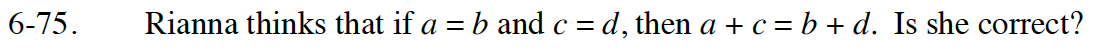Home > GB8I > Chapter cc46 > Lesson cc46.2.3 > Problem6-75

6-75.

Rianna thinks that if a = b and c = d, then a + c = b + d. Is she correct? Homework Help ✎If you add the same amount to both sides of the equals sign, then the equation remains true.

Adding c to one side is the same as adding d to the other because c and d are equal.

Therefore, the equation ( a + c = b + d ) is correct.